SEARCH HOMEMath Central Quandaries & QueriesQuestion from Sakeena, a student: (2^2*3)^x+1/2^2x*3xHi Sakeena.

It is always difficult to interpret what people mean when they write complex algebraic (or even arithmetic) expressions without many parentheses, because usually they mean to group terms differently than the order of operations dictates.

By following the order of operations on what you have written (2^2*3)^x+1/2^2x*3x, we get the following: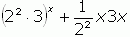After brackets, exponentiation is processed, then division and multiplication, finally addition and subtraction, hence the acronym BEDMAS was invented.

I will show you how to simplify this, but I think you probably meant one of the following: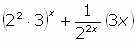which should be written as (2^2*3)^x+1/2^(2x)*3x or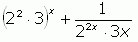which should be written as (2^2*3)^x+1/((2^(2x))*3x) or pe.rhaps even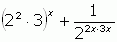which should be written as (2^2*3)^x+1/2^(2x*3x).

However,can be simplified, and that's mathematically what you asked for, so here is what I would do to simplify it.

First, evaluate the small constants and collect the two factors of x, making a fraction: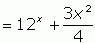This is a mixed fraction and it is in rather simple terms already, but I prefer to use improper fractions rather than mixed fractions, so I would create a common denominator of 4 and simplify it to: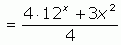Which is where I'd leave it.

If you intended one of the other intepretations (or one that I didn't mention at all), please keep the order of operations in mind and use brackets (parentheses) to group terms where necessary.

Cheers,
Stephen La Rocque.Math Central is supported by the University of Regina and The Pacific Institute for the Mathematical Sciences.